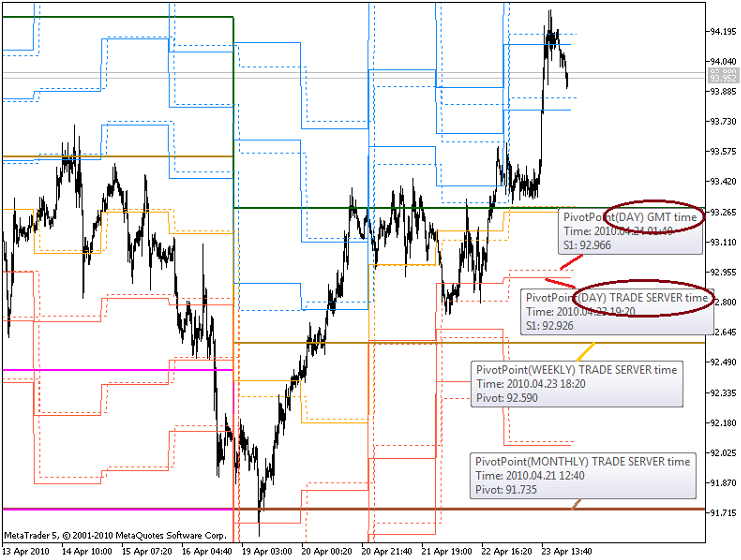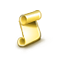Interesting script?
So post a link to it -
let others appraise it
You liked the script? Try it in the MetaTrader 5 terminal# PivotPointUniversal - indicator for MetaTrader 5

|
Views:
41770
Rating:
Published:
2010.04.26 10:46
Updated:
2016.11.22 07:32

The indicator plots Pivot levels for all the available history. It doesn't uses any objects.

There are 5 variants of Pivot levels is supported. There are 3 calculation periods: daily, weekly, monthly. For the daily pivot levels it's possible to specify the GMT time shift.

The formulae, used for the calculation, are:

PIVOT_CLASSIC

Pivot (P) = (H + L + C) / 3
Resistance (R1) = (2 * P) - L      Support (S1) = (2 * P) - H
R2 = P + H - L                           S2 = P - H + L
R3 = H + 2 * (P - L)                   S3 = L - 2 * (H - P)

PIVOT_FIBONACCI

Pivot (P) = (H + L + C) / 3
Resistance (R1) = P + 0.382(H – L)      Support (S1) =  P – 0.382(H – L)
R2 = P + 0.618*(H – L)                       S2 = P – 0.618*(H – L)
R3 = H + *(H – L)                             S3 = L - (H – L)

PIVOT_DEMARK

If C < O Then X = H + 2 * L + C;
If C > O Then X = 2 * H + L + C;
If C = O Then X = H + L + 2 * C;
Resistance (R1) = X / 2 - L; Support (S1) = X / 2 - H

PIVOT_CAMARILLA

R4 = (H - L) X 1.1 / 2 + C    R3 = (H - L) X 1.1 / 4 + C    R2 = (H - L) X 1.1 / 6 + C    R1 = (H - L) X 1.1 / 12 + C
S1 = C - (H - L) X 1.1 / 12   S2 = C - (H - L) X 1.1 / 6     S3 = C - (H - L) X 1.1 / 4     S4 = C - (H - L) X 1.1 / 2

PIVOT_WOODIES

Pivot (P) = (H + L + 2 X C) / 4
Resistance (R1) = (2 X P) - L         Support (S1) = (2 X P) - H
R2 = P + H - L                              S2 = P - H + LAt the image there are 4 indicators of PIVOT_CLASSIC with the different parameters are presented. The first parameter (GMT shift) is actual for the daily Pivots only.

If you have found any errors, please report at Forum and we will fix them ;)

Translated from Russian by MetaQuotes Software Corp.
Original code: https://www.mql5.com/ru/code/102Murrey Math Lines (support and resistance)

The indicator plots Murrey Math Lines for all available history, it doesn't uses any objects.ZeroLag MACD

The ZeroLag MACD indicator shows the histogram with color of the previous bar.Simple MA Expert Advisor

Something for those who wants to try out new Strategy Tester and don't have any EA.Clipboard

The script gets contents from the Windows Clipboard.